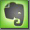Pixels / Resolution / dpi

General

dpi (dots per inch) is the number of dots per inch in a printed image in one dimension (horizontal or vertical). A horizontal print resolution of 150dpi represents 150 individual dots per printed inch horizontally (actually only one single dot 'line'. A general print resolution of 150dpi represents 150 dots horizontally per inch and 150 dots vertically per inch, resulting in 22.500 dots per square inch (150 *150).

In digital images pixel and dots are often but not necessarily identical.

Image Resolution Calculations

With a given resolution of pixels per inch the number of neccessary pixels for a certain printed image format can be calculated by:

number of pixels (width) = image width [cm] * print resolution [dpi]
number of pixels (height) = image height [cm] * print resolution [dpi]

Example: image size 13cm x 18cm with the resolution of 300 dpi:

(13cm / 2,54) * 300dpi = 1535px      (2,54 is the conversion from cm to inch!)
(18cm / 2,54) * 300dpi = 2126px

Total number of pixels = 1535 * 2126 = 3.263.410 pixels

For a given number of pixels the image size for a certain resolution can be calculated:

number of pixels of image width / resolution [dpi] * (cm-inch conversion) = image width [cm]
number of pixels of image height / resolution [dpi] * (cm-inch conversion) = image width [cm]

Example: image width 1535 pixels, image height 2126 pixels

(1535px / 300dpi) * 2,54 = 13cm
(2126px / 300dpi) * 2,54 = 18cm